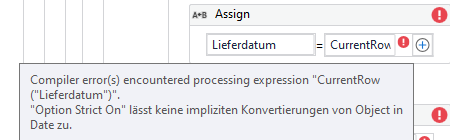# Assign Column as type date from datatable (source excel)

Hi everyone,

so i have an excel file which is implemented in UiPath as a datatable.
From this datatable I use for each activity to calculate for each row the difference of days of two columns.

So I got column one (“Lieferdatum”) and column two (“Umterminierungsdatum”). Both of them are type Date in Excel.

I know want to calculate the difference of days for these two columns.

Sadly the calculation isn’t working yet. My Assign activities look like that:

``````var. Lieferdatum = CurrentRow("Lieferdatum")
var. Umterminierungsdatum = CurrentRow("Umterminierungsdatum")
``````

`var. CalculateVariance = DateDiff(DateInterval.Day, Lieferdatum, Umterminierungsdatum)`

But the assign activities give me the following errors:
“Doesn’t allow conversion from Object to Date”How is it possible to remove these errors or to calculate the differenc properly?

Regards Laura

@laura.groeger

You need to convert them to date.Try as below

`var. Lieferdatum = cdate(CurrentRow("Lieferdatum").ToString) var. Umterminierungsdatum = Cdate(CurrentRow("Umterminierungsdatum").ToString)`

And change the datatupe of your liefrdatum and umte… to datetime

Hope this helps

Cheers

1 Like

Hey

as they are object type and you assingin to a string variable, your should convert to Strinmg like this

``````CurrentRow("Lieferdatum").ToString
``````

Regards

1 Like

@laura.groeger

Basically thats is the reason we are converting to date format so that you can calculate the difference as needed…

Are you still facing some error?

Cheers

You can use the below code to calculate the difference in days between the two dates.

Cdate function is use to convert string into date variable.
I am assuming date format in excel cell is ‘dd-MM-yyyy’
int_days is an integer variable to store number of days.
int_numOfDays= DateDiff(DateInterval.Day, DateVar1, DateVar2)

``````int_days = DateDiff(DateInterval.Day, DateTime.ParseExact(Lieferdatum.ToString, "dd-MM-yyyy",System.Globalization.CultureInfo.InvariantCulture),DateTime.ParseExact(Umterminierungsdatum.ToString, "dd-MM-yyyy", System.Globalization.CultureInfo.InvariantCulture)
``````

Let me know if you need any clarification on the same.

Regards,
Ashutosh Gupta

1 Like

Hi Anil,

it works now! Thanks a lot1 Like

This topic was automatically closed 3 days after the last reply. New replies are no longer allowed.